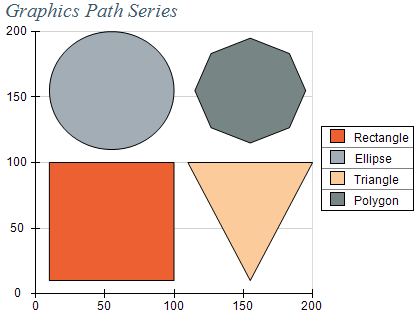﻿ Graphics Path
Nevron .NET Vision
Chart for .NET / User's Guide / Chart Types / Graphics Path / Graphics Path

In This Topic
Graphics Path
In This Topic

A Graphics Path chart displays an arbitrary figure defined by lines and bezier segments. The following figure shows several graphics path series inside a chart that are used to draw figures at different scale coordinates:Figure 1.

Creating a Polar Range series

Graphics Path series are represented by the NGraphicsPathSeries type. An instance of this type must be added to the series collection of a Cartesian Chart).

C#
Copy Code
```NChart chart = nChartControl1.Charts;
NGraphicsPathSeries graphicsPathSeries = new NGraphicsPathSeries();
```
Visual Basic
Copy Code
```Dim chart As NChart = NChartControl1.Charts(0)
Dim graphicsPathSeries As New NGraphicsPathSeries()
```
Passing Data

Once the series is created you can add some data in it. The shape represented by the graphics path is contained inside a NGraphicsPath object exposed from the GraphicsPath property of the series. The following code snippets show how to draw different shapes using this series:

C#
Copy Code

// draw rectangle
NGraphicsPathSeries rectangleSeries = new NGraphicsPathSeries();
NGraphicsPath rectanglePath = new NGraphicsPath();
rectangleSeries.GraphicsPath = rectanglePath;

// draw ellipse
NGraphicsPathSeries ellipseSeries = new NGraphicsPathSeries();
NGraphicsPath ellipsePath = new NGraphicsPath();
ellipseSeries.GraphicsPath = ellipsePath;

// draw triangle
NGraphicsPathSeries triangleSeries = new NGraphicsPathSeries();
NGraphicsPath trianglePath = new NGraphicsPath();
trianglePath.StartFigure(0.0, 0.0);
trianglePath.LineTo(0.5, 1.0);
trianglePath.LineTo(1.0, 0.0);
trianglePath.CloseFigure();
triangleSeries.GraphicsPath = trianglePath;

Visual Basic
Copy Code

' draw rectangle
Dim rectangleSeries As New NGraphicsPathSeries()
Dim rectanglePath As New NGraphicsPath()
rectangleSeries.GraphicsPath = rectanglePath

' draw ellipse
Dim ellipseSeries As New NGraphicsPathSeries
Dim ellipsePath As New NGraphicsPath()
ellipseSeries.GraphicsPath = ellipsePath